# Difference between revisions of "Shortest Path Faster Algorithm"

The Shortest Path Faster Algorithm (SPFA) is a single-source shortest paths algorithm whose origin is unknown[see references]. It is similar to Dijkstra's algorithm in that it performs relaxations on nodes popped from some sort of queue, but, unlike Dijkstra's, it is usable on graphs containing edges of negative weight, like the Bellman-Ford algorithm. Its value lies in the fact that, in the average case, it is likely to outperform Bellman-Ford (although not Dijkstra's). In theory, this should lead to an improved version of Johnson's algorithm as well.

## The algorithm

The algorithm works by repeatedly selecting a vertex and using it to relax, if possible, all of its neighbors. If a node was successfully relaxed, then it might in turn be necessary to use it to relax other vertices, and hence it is marked for consideration also. Once there are no vertices left to be considered, the algorithm terminates. Note that a vertex might be considered several times during the course of the algorithm. The usual implementation strategy is to use a queue to hold the vertices that might be considered next.

#### Pseudocode

input G,v
for each u ∈ V(G)
let dist[u] = ∞
let dist[v] = 0
let Q be an initially empty queue
push(Q,v)
while not empty(Q)
let u = pop(Q)
for each (u,w) ∈ E(G)
if dist[w] > dist[u]+wt(u,w)
dist[w] = dist[u]+wt(u,w)
if w is not in Q
push(Q,w)


Note: We use an array of boolean flags to keep track of which vertices are currently in the queue.

#### Proof of correctness

We will prove that the algorithm never computes incorrect shortest path lengths.

Lemma: Whenever the queue is checked for emptiness, any vertex currently capable of causing relaxation is in the queue.
Proof: We want to show that if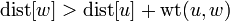$\mathrm{dist}[w] > \mathrm{dist}[u] + \operatorname{wt}(u,w)$ for any two vertices$u$ and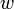$w$ at the time the condition is checked,$u$ is in the queue. We do so by induction on the number of iterations of the loop that have already occurred. First we note that this certainly holds before the loop is entered: if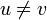$u \neq v$, then relaxation is not possible; relaxation is possible from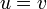$u = v$, and this is added to the queue immediately before the while loop is entered. Now, consider what happens inside the loop. A vertex$u$ is popped, and is used to relax all its neighbors, if possible. Therefore, immediately after that iteration of the loop,$u$ is not capable of causing any more relaxations (and does not have to be in the queue anymore). However, the relaxation by$u$ might cause some other vertices to become capable of causing relaxation. If there exists some$x$ such that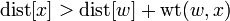$\mathrm{dist}[x] > \mathrm{dist}[w] + \operatorname{wt}(w,x)$ before the current loop iteration, then$w$ is already in the queue. If this condition becomes true during the current loop iteration, then either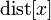$\mathrm{dist}[x]$ increased, which is impossible, or$\mathrm{dist}[w]$ decreased, implying that$w$ was relaxed. But after$w$ is relaxed, it is added to the queue if it is not already present.$_{_\blacksquare}$
Corollary: The algorithm terminates when and only when no further relaxations are possible.
Proof: If no further relaxations are possible, the algorithm continues to remove vertices from the queue, but does not add any more into the queue, because vertices are added only upon successful relaxations. Therefore the queue becomes empty and the algorithm terminates. If any further relaxations are possible, the queue is not empty, and the algorithm continues to run.$_{_\blacksquare}$

The algorithm fails to terminate if negative-weight cycles are reachable from the source. See here for a proof that relaxations are always possible when negative-weight cycles exist. In a graph with no cycles of negative weight, when no more relaxations are possible, the correct shortest paths have been computed (proof). Therefore in graphs containing no cycles of negative weight, the algorithm will never terminate with incorrect shortest path lengths.